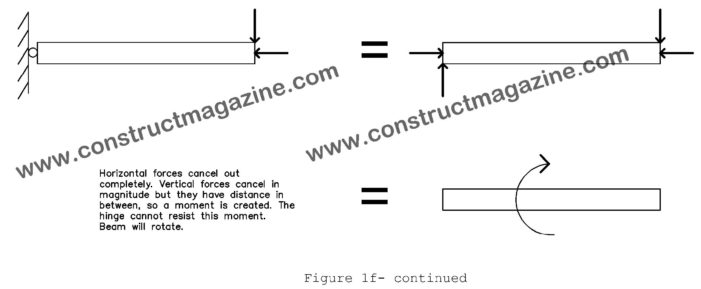## Equlibrium Concept, Action / ReactionAs Sir Isaac Newton discovered centuries ago, a force, (if unbalanced by an equal reaction acting on the opposite direction) will create an acceleration on the body, as in F=ma, where F is the force in newtons, m is the mass in kg and a is acceleration in m/s2. Same is true for turning effects. […]